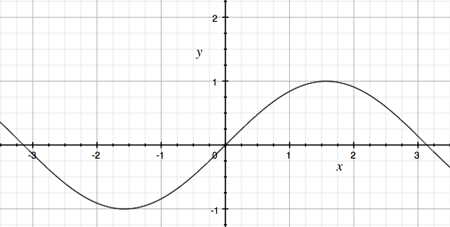SEARCH HOMEMath Central Quandaries & QueriesQuestion from anamika, a student: number of solutions of sinx = x^2 + x +1 graphically?Hi Anamika,

I would first sketch $y = \sin x.$Now consider $y = x^2 + x + 1.$ The function is a quadratic so the graph is a parabola which either opens upwards or downwards. Which is it?

Plot a few points on the graph of $y = x^2 + x + 1,$ for example $x = 0, x = 1 \mbox{ and } x = -1.$ Sketch the graph of $y = x^2 + x + 1.$ How many points lie on both graphs?

PennyMath Central is supported by the University of Regina and The Pacific Institute for the Mathematical Sciences.